Reach Us+44-1522-440391
Meander Graphs and Frobenius Seaweed Lie Algebras II | OMICS International
Journal of Generalized Lie Theory and Applications
All submissions of the EM system will be redirected to Online Manuscript Submission System. Authors are requested to submit articles directly to Online Manuscript Submission System of respective journal.

# Meander Graphs and Frobenius Seaweed Lie Algebras II

Vincent Coll1*, Matthew Hyatt2, Colton Magnant3 and Hua Wang3

1Department of Mathematics, Lehigh University, Bethlehem, PA, USA

2Mathematics Department, Pace University, Pleasantville, NY, USA

3Department of Mathematical Sciences, Georgia Southern University, Statesboro, GA, USA

Corresponding Author:
Vincent Coll
Department of Mathematics
Lehigh University, Bethlehem
PA, USA
Tel: 610-758-3732
E-mail: [email protected]

Received date: March 14, 2015 Accepted date: July 26, 2015 Published date: July 29, 2015

Citation: Coll V, Hyatt M, Magnant C, Wang H (2015) Meander Graphs and Frobenius Seaweed Lie Algebras II. J Generalized Lie Theory Appl 9: 227. doi:10.4172/1736-4337.1000227

Copyright: © 2015 Coll V, et al. This is an open-access article distributed under the terms of the Creative Commons Attribution License, which permits unrestricted use, distribution, and reproduction in any medium, provided the original author and source are credited.

Visit for more related articles at Journal of Generalized Lie Theory and Applications

#### Abstract

We provide a recursive classification of meander graphs, showing that each meander is identified by a unique sequence of fundamental graph theoretic moves. This sequence is called the meander’s signature and can be used to construct arbitrarily large sets of meanders, Frobenius or otherwise, of any size and configuration. In certain special cases, the signature is used to produce an explicit formula for the index of seaweed Lie subalgebra of sl(n) in terms of elementary functions.

#### Keywords

Biparabolic; Frobenius; Lie algebra; Meander; Seaweed algebra

#### Introduction

Dergachev et al.  introduced meanders as planar graph representations of bi-parabolic, or seaweed, subalgebras of sl(n) and also provided a combinatorial method of computing the index of such seaweeds from the number and type of the connected components of their associated meander graphs. Of particular interest are those seaweed algebras whose associated meander graph consists a single path. Such algebras have index zero. More generally, algebras with index zero are called Frobenius and have been extensively studied in the context of invariant theory [2-5] and more recently from the point of view of quantum group theory .

Extending the work of Elashvilli, Coll et al. [2,6] showed that a seaweed of type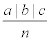was Frobenius precisely when gcd (a + b, b + c)=1. However, the methods used there do not extend to seaweeds with more than four blocks. Also, the question of what the index actually is for the non-Frobenius four block case was left unaddressed. We take up these questions in this follow-up note, by first providing a recursive classification of meander graphs, showing that each meander is identified by a unique sequence of fundamental graph theoretic moves.

This sequence is called the meander’s signature. The signature provides a fast algorithm for the computation of the index of Lie algebra associated with the meander, and can therefore be used to test any relatively prime conditions based on the type of the meander that might define Frobenius seaweed Lie algebra. In particular, we find that an easy induction on the moves of the signature gives that the index of a seaweed of typeis gcd (a + b, b + c)−1. The signature also yields, with relative ease, new infinite families of Frobenius seaweed Lie algebras of any size and type.

#### Definitions

In this section, we detail the notions of the index of Lie algebra, and seaweed algebras and the meanders associated with them.

Index

Definition 1. Let g be Lie algebra over a field of characteristic zero. For any functional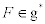one can associate the Kirillov form BF (x, y) = F [x, y] which is skew-symmetric and bilinear. The index of g is defined to be the minimum dimension of the kernel of BF as F ranges over g*. The Lie algebra g is Frobenius if its index is zero.

Seaweed algebras

Definition 2. Let k be an arbitrary field of characteristic 0 and n a positive integer. Fix two ordered partitions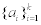and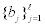of the number n. Let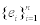be the standard basis in kn. A subalgebra of sl(n) that preserves the vector spaces{Vi = span (e1, . . . , ea1+…+ai )} and {Wj = span (eb1+…+bj+1, ..., en)} is called a seaweed algebra of type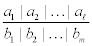due to their suggestive shape when exhibited in matrix form (Given in left hand side of Figure 1).

Remark. A basis free definition is available but not necessary for the present discussion. Also, the notion of seaweed algebra has been extended to reductive algebras by Panychev .

Meanders

Definition 3. To each seaweed algebra, a planar graph, called a meander, can be constructed as follows: Line up n vertices and label them with v1, v2, . . . , vn and partition this set into two ordered partitions, called top and bottom. For each part in the top (likewise bottom) we build up the graph by adding edges in the same way. This involves adding the edge from the first vertex of a part to the last vertex of the same part drawn concave down (respectively concave up in the bottom part case). The edge addition is then repeated between the second vertex and the second to last and so on within each part of both partitions. The parts of these partitions are called blocks. With top blocks of sizes a1, a2, . . . , a and bottom blocks of sizes b1, b2, . . . , bm, we say such a meander has type(Given in right hand side of Figure 1).

#### Recursive Classification and Winding Down

In this section, we show that any meander can be contracted or “Wound Down” to the empty meander through a sequence of graphtheoretic moves; each of which is uniquely determined by the structure of the meander at the time of move application. We find that there are five such moves, only one of which affects the component structure of the graph and is therefore the only move capable of modifying the index of the graph, here defined to be twice the number of cycles plus the number of paths minus one. Dergachev and Kirillov showed that the index of the graph is precisely the index of the associated seaweed subalgebra. Since the sequence of moves which contracts a meander to the empty meander uniquely identifies the graph, we call this sequence the meander’s signature. Although developed independently, we find that the signature is essentially a graph theoretic recasting of Panyushev’s reduction algorithm, which  was used to develop inductive formulas for the index of seaweeds in gl(n). Here these inductive formulas are expressed in terms of elementary functions, which are laid plain by the explicit nature of the signature.

Lemma 4 (Winding Down). If M is a general meander of type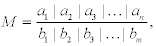the we have the following cases:

1. Flip (F) If a1 < b1, then simply exchange ai for bi to get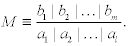2. Component elimination (C(c)) If a1 = b1, then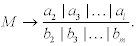3. Block elimination (B) If a1 = 2b1, then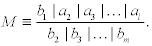4. Rotation contraction (R) If b1 <a1< 2b1, then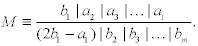5. Pure contraction (P) If a1 > 2b1, then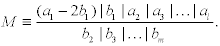Note that the Winding Down moves can be reversed to create a set of “Winding Up” moves, which can be used to build all meanders, Frobenius and otherwise.

Remark. Although not our concern here, one of the major questions for both open and closed meanders is their enumeration. Using a different family of index preserving operators on meanders graphs, Dufflo and Tu have recently developed an approach which can, in certain cases, effect this enumeration via polynomials .

The signature

We call the cases in Lemma 4 moves since they move one meander to another. Notice that in each of these moves except the Flip, the number of vertices in the graph is reduced. Also note that in each move except for the Component Elimination move, the component structure of the meander is maintained. Given a meander, there exists a unique sequence of moves (elements of the set {F, C(c), B, R, P}) which reduce the given meander down to the empty meander. Such a list is called the signature of the meander. Since the only move that changes the component structure is C(c), we get the following corollary, which provides a recursive classification of Frobenius meanders.

Theorem 5 (Recursive classification). A meander is Frobenius if and only if the signature contains no C(c) move except the very last move which must be C(1).

Example: As an example of the signature of a Frobenius meander, considers the meander of type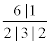This meander has signature PF RBF BF BC(1), the movements for which are demonstrated in the following Figure 2.

Theorem 5 provides a fast algorithm for computing the signature of a meander. Indeed, from the signature, one can read off the index of the meander by simply adding the parameters used in the Component Elimination moves and subtracting 1. That is, if {c1, c2, . . . , cm} are the parameters used in the Component Elimination moves, then the index of the meander equals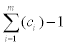So, there is a linear (in the order of the meander) time algorithm for computing the index of the meander.

Index of a Meander with 4 Blocks

The determination of whether seaweed sub algebra is Frobenius usually relies on a combinatorial argument. This section recasts known formulas for computing the index in terms of elementary functions. Theorem 8 provides a new addition to this class of formulas and is a strengthening of a result of Coll et al. .

Theorem 6. A meander of type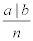has index gcd(a, b) – 1 .

In order to prove the next result, we make repeated use of the following easy fact that follows from the Euclidean Algorithm.

Fact 7. For integers a and b, gcd(a, b)=gcd(a, a + b).

Now, in the style of Theorem 6, we have the following pleasing result.

Theorem 8. A meander of type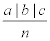(or type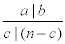) has index gcd(a + b, b + c) - 1.

Certainly an F move has no effect on the index of the meander so we may assume any meander with four blocks is in one of these forms or its flip, whichever is more convenient at the time.

Proof. Let M be a meander with 4 blocks. The proof is by induction on the number of simplified Winding Down moves in the signature of M. Let k be the number of moves in the signature before either a C(c) move or B move is performed. The base of this induction, when k = 0, is provided by Cases 1 and 2. We consider cases based on the first simplified Winding Down move performed in the signature of M. Let M′ be the result of this first Winding Down move.

Case 1. A Component Elimination move C(c) is performed.

In this case, M′ has only two blocks so must look like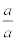This means M must have type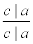Thus, the index of this meander is clearly a + c - 1 while the formula says the index should be gcd(c + a, c + a) − 1 = a + c − 1.

Case 2. A Block Elimination move B is performed.

This case has two subcases. The first is where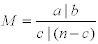and a=2c. Then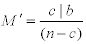By Theorem 6, the index of M′ is gcd(b, c)-1. Since a = 2c, using Fact 7, this value is the same as gcd(a + b, b + c) − 1 and the B move preserves the index, the index of M is gcd(a + b, b + c) − 1 as claimed. The second case is where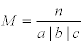so n = 2a and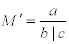By Theorem 6, the index of M′ is gcd(b, c) - 1. Since n=a + b + c=2a, by Fact 7, this value is the same as gcd(a + b, b + c) − 1 and the same argument yields the index of M.

Case 3. A Rotation Contraction move R is performed.

Ifthen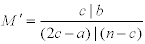By induction, the index of M′ is gcd(c + b, b + 2c − a) − 1. By Fact 7, gcd(c + b, b + 2c − a) − 1 = gcd(a + b, b + c) − 1 and since the index is preserved under the R move, the index of M is as claimed. Ifan identical argument holds.

Case 4. A Pure Contraction move P is performed.

Ifthen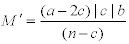By induction, the index of M′ is gcd(a − c, b + c) − 1. By Fact 7, this equals gcd (a + b, b + c) − 1 and since the index is preserved under a P move, the index of M is as claimed. Ifan identical argument holds.

Note that Theorem 6 can be reproven very easily using exactly the same approach. We now have the following easy corollary to Theorem 8, which is the main result .

Theorem 9. A meander of typeor typeis Frobenius if and only if gcd(a + b, b + c) = 1

New infinite families of Frobenius meanders

While known infinite families of Frobenius meanders are few, new such families can, in theory, be developed by routine applications of inductive formulas developed by Panychev . However, we find that, in practice, and in keeping with our approach here, that recognizing if seaweed is Frobenius directly from its block type, via a relatively prime condition, is difficult. To illustrate, we note that while it is known that Frobenius meanders can have an arbitrarily large number of blocks [7,9,10], it is non-trivial to show that they can have arbitrarily many blocks of arbitrarily large size.

Theorem 10. If a is even and gcd(a, b) = 1, then the meander of type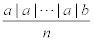is Frobenius.

Remark. The proof relies on a lengthy counting argument. We relegate the proof to Appendix A.

The simplified Winding Down process can be used in cooperation with Theorem 10 to obtain the following more general family of biparabolics:

Corollary 11. If a is even and gcd(a, b) = 1, then the meander of type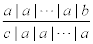is Frobenius where c=b + ka for some integer k.

Proof. Let a, b and c be as given and let ℓ be the number of blocks of size a on the bottom in this meander. By Theorem 10, the meander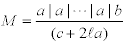is Frobenius (where there are ℓ extra blocks of size a on the top of this meander). To M, we apply an F move followed by ℓ P moves. The resulting meander is then flipped again using the F move to obtain the meander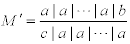Since M was Frobenius, M′ is as well.

#### Conclusion

Following Theorems 6 and 8, one might expect that a Frobenius meander of type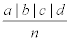can be characterized by a relatively prime condition of the form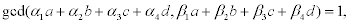where the αi and βj are integer coefficients. Substantial empirical evidence suggests that this is not so. Using the signature, exhaustive simulations have shown that there is no set of integer coefficients, all with absolute value at most ten, which can be used to build such a relatively prime condition. While this in not conclusive, it is compelling. And since the addition of blocks seems to only complicate matters, we are led to conjecture that no single, or even finite set of, relatively prime conditions are sufficient to classify Frobenius meanders with at least five blocks.

Ongoing work

The notion of a signature consisting of a deterministic sequence of index preserving graph theoretic moves can be applied to other families of Lie algebras. A forthcoming note will introduce the notion of a “symplectic meander” from which a finite set of relatively prime conditions can be used to identify Frobenius seaweed subalgebras of sp(2n) in the three and four block case. The so(2n) case seems to be similarly tractable . However, the five block case appears to be a barrier to this “relatively prime” approach in all cases.

#### Appendix - Proof of Theorem 11

In this proof, we will be working with meanders which have multiple top blocks and a single bottom block, so we make use of the following simplified notation: Write a meander of type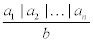as a1|a2|…|an. We also require a preliminary

Lemma 12. For an even integer a and an odd integer b, a meander with k blocks a|a|…|a|b has index m if and only if the meander a|a|… |a|(b + 2a) with the same number of blocks also has index m.

Proof. The proof of this lemma consists of simply showing that any path and cycle structure is preserved as the meander is transformed between the two meanders M and M′ described below. Let A1, A2, . . . , Ak−1 denote the k − 1 blocks of order a (appearing in this order) with A1 = u1, u2, . . . , ua and let B+ denote a block of order b + 2a with vertices v1, v2, . . . , vb+2a. Then all bottom edges of A1 go to the rightmost a vertices of B+, namely the vertices vb+a+1, vb+a+2, . . . , vb+2a. Using the top edges, these vertices are adjacent to the leftmost a vertices of B+, namely the vertices v1, v2, . . . , va.

For each integer i with 1 ≤ i ≤ a/2, we may replace the path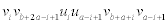with a new edge viva−i+1 where all internal vertices of the path are removed from the meander. Define A′1 to be the vertices v1, v2, . . . , va. and define B to be the vertices va+1, va+2, . . . , vb+a. Note that all the vertices of A1 along with vb+a+1, v b+a+2, . . . , vb+2a have been removed. Call this new graph M′. Then M′ is again a meander, and since we have replaced paths with single edges, all path and cycle structure, and so the index has been preserved. This process can easily be reversed to produce M from M′.

In particular, Lemma 12 means that, when considering whether a general meander of type a| a | . . . | a | b is Frobenius, we may assume b < 2a. Now, call a segment between consecutive vertices in the drawing of a meander an end-segment if there is an edge of the meander connecting the two vertices on either end of this segment. Call a segment a top-endsegment if the segment is an end-segment and the corresponding edge is a top-edge. A segment gets mapped by the meander by following either the bottom edges or the top edges on either side of the segment. By Theorem 8, we may assume there are at least 3 blocks of size a in this meander. If we suppose a=2, then by Lemma 12, we know b ≤ 3. Evidently, this meander is Frobenius. Thus, we may assume a ≥ 4. If there was a cycle, then the Jordan Curve Theorem implies that there exists a segment between vertices that does not map (following edges of the meander) to the exterior face. Since we show that this is not the case, there must not be a cycle in the meander. This proof involves considering the segments between vertices in the meander graph and showing that each top-end-segment must be mapped by the meander to the exterior face. We then consider any segment and show that it either maps to a top-end-segment or to the exterior face.

Intuitively, the proof makes use of the following heuristic: We visualize the meander as an object (much like a shell) with openings in the top between the blocks. If water is poured into these openings, we ask whether the water permeates all areas inside the shell. If so, the meander is Frobenius and if not, it contains a cycle and is therefore not Frobenius.

Label the segments between consecutive vertices of the meander in the natural (drawn) order from 1 up to ka + b − 1 where k is the number of even blocks. Let c=a/2 and note that, since c ≥ 2, c also shares no common factor with b. Then any top-end-segment must be labeled with ωc where ω is an odd positive integer. Furthermore, the exterior face is accessible via any segment labeled ia = 2ic for any positive integer i ≤ k.

For any segment labeled x, following the bottom mapping by the meander yields 2kc+b−x since c = a/2 . We denote this by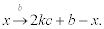When following the top mapping by the meander, we have two cases. If we are in the odd group (of size b), we would map x to 4kc + b − x so we denote this by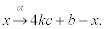Suppose now we map within an even group (of size a). If we start in the ℓth such group, we would map x to (2ℓ − 1)2c − x which is denoted by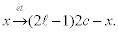These maps will be called arrow maps.

Our first goal is to show that, starting with a top-end-segment labeled ωc and following the mapping by the meander, we would reach the exterior face (represented by a segment labeled 2ic for some positive integer i as above) before ever reaching another top-endsegment. In order to accomplish this, we show that any sequence of these arrow maps would send ωc to 2ic before ever reaching ω′c for some positive odd integer ω′. After the first bottom map, we get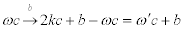for some positive odd integer ω′. Certainly this is not an even multiple of c since b and c share no common factors. We now consider sequences of top maps and bottom maps, called double mappings, by the meander. This gives us two cases: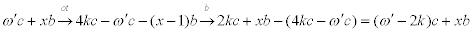or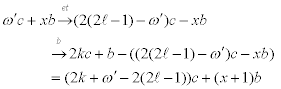where x is a positive integer.

In either case, the result is an odd multiple of c plus a multiple of b where the multiple of b never decreases and increases by at most one after double mapping. Since this double mapping can be repeated, we see that ωc will only map to ω′c + mb where ω′ is odd and m is a positive integer. Suppose we have chosen m to be the smallest positive integer with ω′c + mb=dc where d is a positive integer. Our goal is to show that d is even.

Since c and b share no common factor, we know b|(d − ω′) and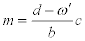If d is odd, then d − ω′ is even, making m even. Then, if we consider m as opposed to m, we see that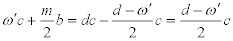which is an integer multiple of c, contradicting the minimality of the choice of m. Thus, d is even and every top-end-segment maps to the exterior face of the meander.

Our next goal is to show that every segment maps to either a top-end-segment or the exterior face (in fact both are true). This will complete the proof that there is no cycle in the meander and establish that the meander is Frobenius.

Consider an arbitrary segment and define an operation to be first a bottom map and then a top map. Note that since the total number of vertices is odd, there is no bottom-end-segment so an operation is well defined for all segments unless the bottom map arrives at a top-endsegment or the exterior face. We will call such operations terminating since the operation cannot actually be completed as defined.

As shown above, any operation where the top map does not occur within the last (odd) block must add b plus an integer multiple of c to the label of the segment. Since we may suppose b<2a by Lemma 12 and we have assumed that there are at least 3 blocks of size in this meander, every operation in which the top map occurs within the last block must be followed immediately by an operation in which the top map does not occur within the last block. Thus, any sequence of operations must add an integer multiple of c along with a strictly increasing positive integer multiple of b to the label of the segment. Since b shares no common factors with c (or a), this sequence of operations must terminate at either a top-end-segment or the exterior face, namely a segment label which is a multiple of c. Thus, there can be no cycle in the meander so the meander is Frobenius.

#### References

Select your language of interest to view the total content in your interested language

### Article Usage

• Total views: 12411
• [From(publication date):
July-2015 - Aug 21, 2019]
• Breakdown by view type
• HTML page views : 8533
• PDF downloads : 3878

## Post your commentCan't read the image? click here to refresh
###### Peer Reviewed Journals

Make the best use of Scientific Research and information from our 700 + peer reviewed, Open Access Journals

International Conferences 2019-20

Meet Inspiring Speakers and Experts at our 3000+ Global Annual Meetings

Top